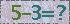2019年JOS入选“中国科技期刊卓越行动计划”
J. Semicond. > Volume 34 > Issue 8 > Article Number: 085015

# A 14-bit 100-MS/s CMOS pipelined ADC with 11.3 ENOB

Ke Wang , , Chaojie Fan , Jianjun Zhou and Wenjie Pan

Abstract: This paper demonstrates a 14-bit 100-MS/s pipelined analog-to-digital converter (ADC) in 0.18 μm CMOS process with a 1.8 V supply voltage. A fast foreground digital calibration mechanism is employed to correct capacitor mismatches. The ADC implements an SHA-less 3-bit front-end to reduce the size of the sampled capacitor. The presented ADC achieves a 70.02 dB signal-to-noise distortion ratio (SNDR) and an 87.5 dB spurious-free dynamic range (SFDR) with a 30.7 MHz input signal, while maintaining over 66 dB SNDR and 76 dB SFDR up to 200 MHz input. The power consumption is 543 mW and a total die area of 3×4 mm2 is occupied.

Abstract: This paper demonstrates a 14-bit 100-MS/s pipelined analog-to-digital converter (ADC) in 0.18 μm CMOS process with a 1.8 V supply voltage. A fast foreground digital calibration mechanism is employed to correct capacitor mismatches. The ADC implements an SHA-less 3-bit front-end to reduce the size of the sampled capacitor. The presented ADC achieves a 70.02 dB signal-to-noise distortion ratio (SNDR) and an 87.5 dB spurious-free dynamic range (SFDR) with a 30.7 MHz input signal, while maintaining over 66 dB SNDR and 76 dB SFDR up to 200 MHz input. The power consumption is 543 mW and a total die area of 3×4 mm2 is occupied.

References:

  Ali A, Morgan A, Dillon C. A 16 b 250 MS/s IF-sampling pipelined A/D converter with background calibration[J]. IEEE J Solid-State Circuits, 2010, 45: 292.  Lewis S H, Fetterman H S, Fross G F. A 10-b 20-M samples/s analog-to-digital converter[J]. IEEE J Solid-State Circuits, 1992, 27(3): 351. doi: 10.1109/4.121557  Chang D Y. Design techniques for a pipelined ADC without using a front-end sample-and-hold amplifier[J]. IEEE Trans Circuits Syst Ⅰ:Regular Papers, 2004, 51(11): 2123.  Lewis S H, Gray P R. A pipelined 5-Msamples/s 9-bit analog-to-digital converter[J]. IEEE J Solid-State Circuits, 1987, 22(6): 599.  Devarajan S, Singer L, Kelly D. A 16-bit, 125 Ms/s, 385 mW, 78.7 dB SNR CMOS pipeline ADC[J]. IEEE J Solid-State Circuits, 2009, 44(12): 3305.  Chiu Y, Grap P, Nikolic B. A 14-b 12-Ms/s CMOS pipeline ADC with over 100-dB SFDR[J]. IEEE J Solid-State Circuits, 2004, 39: 2139.  Razavi B. Design of analog CMOS integrated circuit[J]. International Editions, 2000.  Mehr I, Singer L. A 55-mW, 10-bit, 40-Msample/s Nyquist-rate CMOS ADC[J]. IEEE J Solid-State Circuits, 2000, 35(3): 318. doi: 10.1109/4.826813  Karanicolas A N, Lee H S, Bacrania K L. A 15-b 1-Msample/s digitally self-calibrated pipeline ADC[J]. IEEE J Solid-State Circuits, 1993, 28(12): 1207. doi: 10.1109/4.261994  Cai Hua, Li Ping, Cen Yuanjun. A 14-bit 80 MS/s CMOS ADC with 84.8 dB SFDR and 72 dB SNDR[J]. Journal of Semiconductors, 2012, 33(2): 025012.  Zhao Lei, Yang Yintang, Zhu Zhangming. SHA-less architecture with enhanced accuracy for pipelined ADC[J]. Journal of Semiconductors, 2012, 33(2): 025010.  Luo L, Lin K, Cheng L. A digitally calibrated 14-bit linear 100-MS/s pipelined ADC with wideband sampling frontend[J]. IEEE ESSCIRC, 2009: 472.  Ali A M A, Dillon C, Sneed R. A 14-bit 125 MS/s IF/RF sampling pipelined ADC with 100 dB SFDR and 50 fs jitter[J]. IEEE J Solid-State Circuits, 2006, 41(8): 1848.
  Ali A, Morgan A, Dillon C. A 16 b 250 MS/s IF-sampling pipelined A/D converter with background calibration[J]. IEEE J Solid-State Circuits, 2010, 45: 292.  Lewis S H, Fetterman H S, Fross G F. A 10-b 20-M samples/s analog-to-digital converter[J]. IEEE J Solid-State Circuits, 1992, 27(3): 351. doi: 10.1109/4.121557  Chang D Y. Design techniques for a pipelined ADC without using a front-end sample-and-hold amplifier[J]. IEEE Trans Circuits Syst Ⅰ:Regular Papers, 2004, 51(11): 2123.  Lewis S H, Gray P R. A pipelined 5-Msamples/s 9-bit analog-to-digital converter[J]. IEEE J Solid-State Circuits, 1987, 22(6): 599.  Devarajan S, Singer L, Kelly D. A 16-bit, 125 Ms/s, 385 mW, 78.7 dB SNR CMOS pipeline ADC[J]. IEEE J Solid-State Circuits, 2009, 44(12): 3305.  Chiu Y, Grap P, Nikolic B. A 14-b 12-Ms/s CMOS pipeline ADC with over 100-dB SFDR[J]. IEEE J Solid-State Circuits, 2004, 39: 2139.  Razavi B. Design of analog CMOS integrated circuit[J]. International Editions, 2000.  Mehr I, Singer L. A 55-mW, 10-bit, 40-Msample/s Nyquist-rate CMOS ADC[J]. IEEE J Solid-State Circuits, 2000, 35(3): 318. doi: 10.1109/4.826813  Karanicolas A N, Lee H S, Bacrania K L. A 15-b 1-Msample/s digitally self-calibrated pipeline ADC[J]. IEEE J Solid-State Circuits, 1993, 28(12): 1207. doi: 10.1109/4.261994  Cai Hua, Li Ping, Cen Yuanjun. A 14-bit 80 MS/s CMOS ADC with 84.8 dB SFDR and 72 dB SNDR[J]. Journal of Semiconductors, 2012, 33(2): 025012.  Zhao Lei, Yang Yintang, Zhu Zhangming. SHA-less architecture with enhanced accuracy for pipelined ADC[J]. Journal of Semiconductors, 2012, 33(2): 025010.  Luo L, Lin K, Cheng L. A digitally calibrated 14-bit linear 100-MS/s pipelined ADC with wideband sampling frontend[J]. IEEE ESSCIRC, 2009: 472.  Ali A M A, Dillon C, Sneed R. A 14-bit 125 MS/s IF/RF sampling pipelined ADC with 100 dB SFDR and 50 fs jitter[J]. IEEE J Solid-State Circuits, 2006, 41(8): 1848.

## GET CITATION

K Wang, C J Fan, J J Zhou, W J Pan. A 14-bit 100-MS/s CMOS pipelined ADC with 11.3 ENOB[J]. J. Semicond., 2013, 34(8): 085015. doi: 10.1088/1674-4926/34/8/085015.

Export: BibTex EndNote

## Article Metrics

Article views: 1246 Times PDF downloads: 28 Times Cited by: 0 Times

## History

Manuscript received: 15 January 2013 Manuscript revised: 06 March 2013 Online: Published: 01 August 2013

Code:*验证码错误